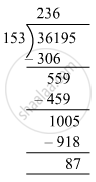# Divide, and find out the quotient and remainder. Check your answer. 36195 ​÷ 153 - Mathematics

Fill in the Blanks

36195 ​÷ 153

#### SolutionQuotient = 236 and Remainder = 87
Check: Divisor × Quotient + Remainder = 153 × 236 + 87
= 36195
= Dividend
∴ Dividend= Divisor × Quotient +Remainder

Is there an error in this question or solution?

#### APPEARS IN

RS Aggarwal Class 6 Mathematics
Chapter 3 Whole Numbers
Exercise 3E | Q 2.3 | Page 56